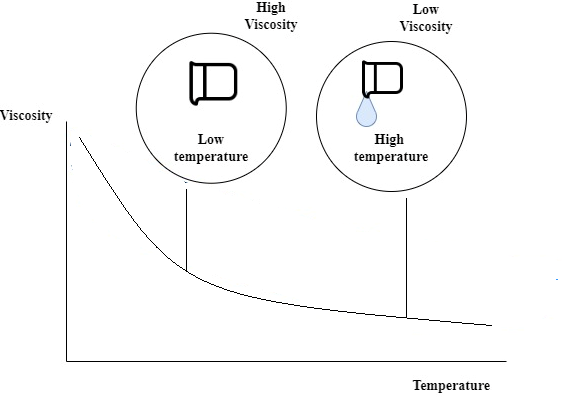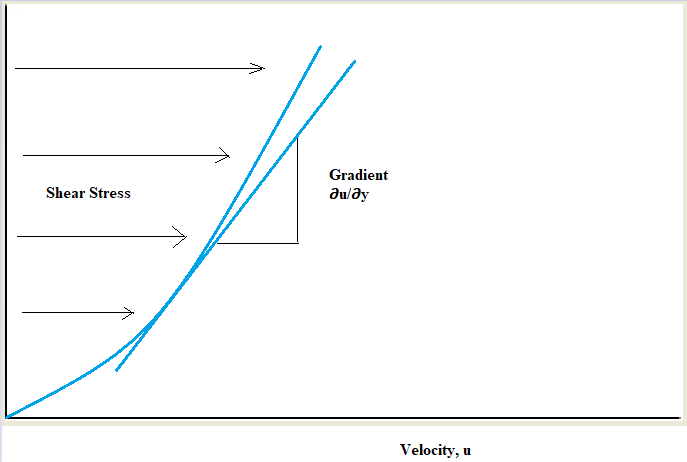# Relation Between Viscosity and Density

## Introduction

Viscosity is used for the measurement of the thinness and thickness of the fluid. On the other hand, density measures the space between two particles of a fluid. Generally, with the increase in temperature, the particles of the fluid fall apart. Thereby, resulting in a decrease in fluid density. Decreases in fluid density affect the viscosity of the liquid. The low density of fluid results in a lowering of the viscosity of the fluid and thereby transforming fluid into a less viscous fluid. The viscosity and density is an essential factor as they help to compare different fluids. The fluids that are more viscous will flow slower than the less viscous fluids.

## Viscosity and Density: Difference

Temperature plays an important role in the viscosity and density of particle fluid. The density of the fluid decreases when the temperature increases and thereby resulting in less viscosity of the fluid.Viscosity and dentistry are one of the two most important phenomena in physics. There are some differences between density and viscosity.

Viscosity Density
Viscosity is generally defined by the measurement of the resistance of a fluid that is deformed by either tensile stress or shear stress Density generally refers to the mass per unit volume
Viscosity is the measurement of molecule shapes and inter-molecular forces Density measures the molecular weight of a composition.
Viscosity generally changes quickly Density changes slightly with the temperature.

## Dynamic Viscosity Measurement

Viscosity is one of the important factors of the fluid. Viscosity is used as an indicator of the quality by the consumers. The thicker liquid is considered as more superior to the thinner product. The rotational viscometer is one of the popular types of instruments that are used for the measurement of the dynamic viscosity. Viscosity is usually evaluated by the measurement of the torque or force which is essential for the rotation of the probe. The rotational viscometer is very useful to measure non-Newtonian liquids (Kassem Çamur & Bennur, 2018).

For example, if applied force can be increased, the viscosity of the non-Newtonian liquids also increases. If a probe moves within the liquid, the rotational viscometer synthesizes the tuning speed of it. According to da Silva et al. (2022), on the other hand, it is also found that in some other non-Newtonian liquids with the enhancement of the applied force, the reduction of viscosity happens.Dynamic viscosity of the fluid generally refers to the measurement of the resistance to flow while an external force has been applied. The SI physical unit of dynamic viscosity ($\mathrm{\mu}$) is Pascal-second (Pa s) that is identical to 1 kg $\mathrm{m^{-1}s^{-1}}$. Physical unit of dynamic viscosity in the centimeter gram second system of units (cgs) is poise (P). It is commonly expressed generally in ASTM standards.

## Kinematic Viscosity Measurement

The kinematic viscosity is the atmospheric variable. Area and time are two important factors on which the units of the kinematic viscosity are generally established. As opined by Pham et al. (2018), there are a few methods that are generally used to measure the Kinematic Viscosity of a particular fluid. The most popular method that is used in the measurement of Kinematic Viscosity is by ascertaining the time that it takes for the fluid which flows through the capillary tube. The time generally converted directly to the kinematic viscosity Centistokes (cSt) is the unit to measure the kinematic viscosity (Pham et al. 2018). The SI unit of the Kinematic viscosity is m$\mathrm{^{2}}$ s-1. Stokes (St) is the physical unit of kinematic viscosity.

## The Equation of the Kinematic Viscosity

Physic not only depends on the mere theoretical description but it also includes the mathematical equations for a better understanding. The equation is mentioned below

v = n/p

Here,

• V = Kinematic viscosity

• n = Absolute viscosity/ Dynamic viscosity

• P = Density

There are three types of units that are used to measure Kinematic Viscosity.

Unit Types of unit
SI unit Kg/ms or N.s/m$\mathrm{^{2}}$
CGS Unit gm/cm.sec or Poise
FPS unit Pound or Reyan.s/inchm$\mathrm{^{2}}$

## Relation between Viscosity and Density

There is n such direct relation between viscosity and density. However, these two factors are affected by the changing in the temperature. In liquids with increasing temperatures, the viscosity of the liquid reduces. In liquids velocity generally increases with the increase of the density (Bae & Halloran, 2019). According to the kinetic theory gases must be proportional to the square root of absolute temperature.

For instance, we can take the example of honey. During the winter season, honey consists of high density as it solidifies and forms a solid-state. The particles are attached to this particular condition. When the same honey is kept under the sun, it melts. So, here is one thing that should be noted carefully.

According to Balla et al. (2020), under the high temperature, the inter-atomic particles form some distance and the friction between the layers of honey reduces while pouring it into the other vessel. These scenarios clearly describe the two parameters that are mentioned below.

• The difference between Viscosity and density

• The relation between Viscosity and density.

## Conclusion

The viscosity and density are the two important factors that determine the characteristics of the fluid. These two factors help to measure the type and nature of the fluid. These two factors are dependent on the changing the temperatures.

## FAQs

Q.1. What is viscosity?

Ans. Viscosity is one of the important characteristic properties of liquids. Viscosity is the measurement of the resistance to the foe that arises due to internal friction between the layers of fluid.

Q.2. How does temperature affect Viscosity?

Ans. The viscosity of the liquid generally decreases when the temperature increases. The molecules hold high kinetic energy and can surpass intermolecular forces to slip past each other between the layers.

Q.3. Which has a lower density than liquids and solids?

Ans. Gases have a lower density than liquids and solids. This happens because the particles of gases are compactly packed in the both states.

Q.4. How pressure is related to density?

Ans. Pressure is straightly proportional to the density in a constant temperature. When the pressure increases, the density also increases.